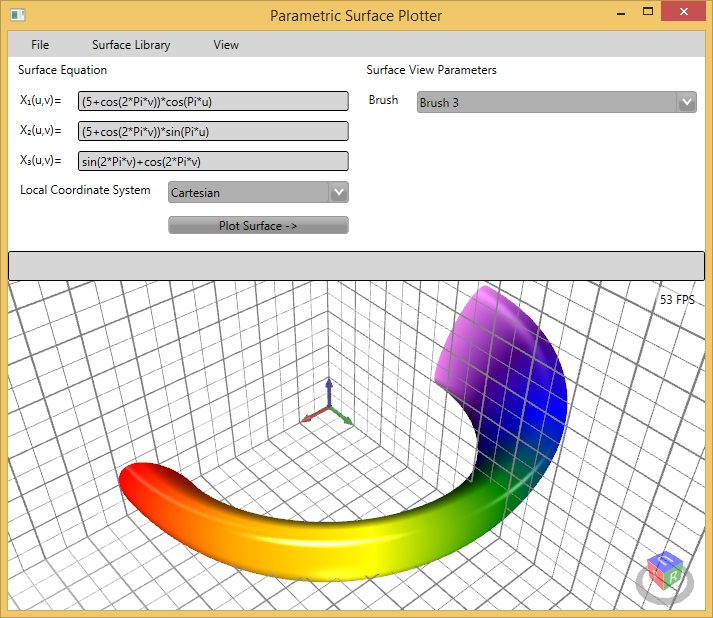ANALYTICS Development library.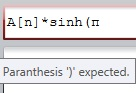ANALYTICS is a source code library for developers. ANALYTICS library contains special classes to work with ‘analytical’ expressions in .NET, Java and Delphi (native) programs – parse expressions, calculate expression values and so on. ANALYTICS library provides easiest way to allow user of your program input data as a formula with syntax checking. You can do analytical calculations and manipulate string expressions inside your program code even – analytical derivative calculations. And finally, you can create ‘analytical calculator’ for your program specific data with minimal code writing.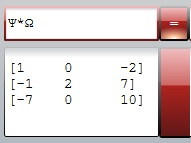Delphi (TMS): https://www.tmssoftware.com/site/tmsanalytics.asp

Documentation:  ANALYTICS_C#_manual.en.pdf  (English)

ANALYTICS_C#_manual.ru.pdf (Russian)

ADVANTAGES of ANALYTICS library: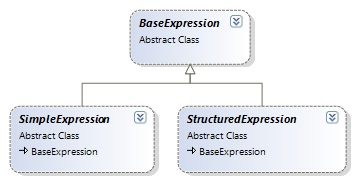1. 100% source code.

2.  Strongly structured class hierarchy.

3. Universal algorithms (working with formulae of any complexity).

4. Analytical derivative calculation.

5.  Many predefined functions.

6.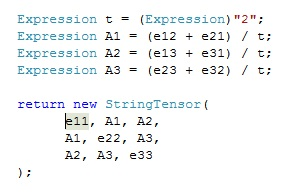Easy to introduce new functions for any argument types.

7. Easy to overload operators for any argument types.

8. Working with Complex numbers.

9. Working with common fractions.

10. Working with 3D vectors and tensors.

11. Working with physical values and units of measurement.

12. Working with indexed data (arrays, matrixes and higher dimensioned data).

13. Statistical analysis of data (base statistics functions, probability distributions).

14 Numerical tools integrated with symbolic features.

Numerical result postprocessor.

FEMPOSTPRO.DEMO.1.0.Setup.rar

Platform: .NET.

Description: Postprocessor for results of mathematical modeling (FEM, BEM i.e.). The program is like ‘analytical calculator’ but works not with real numbers but with the results of physical problems modeling. This feature is realized via ANALYTICS technology, which allows calculating string (formula) expression for any data types, by unified algorithm.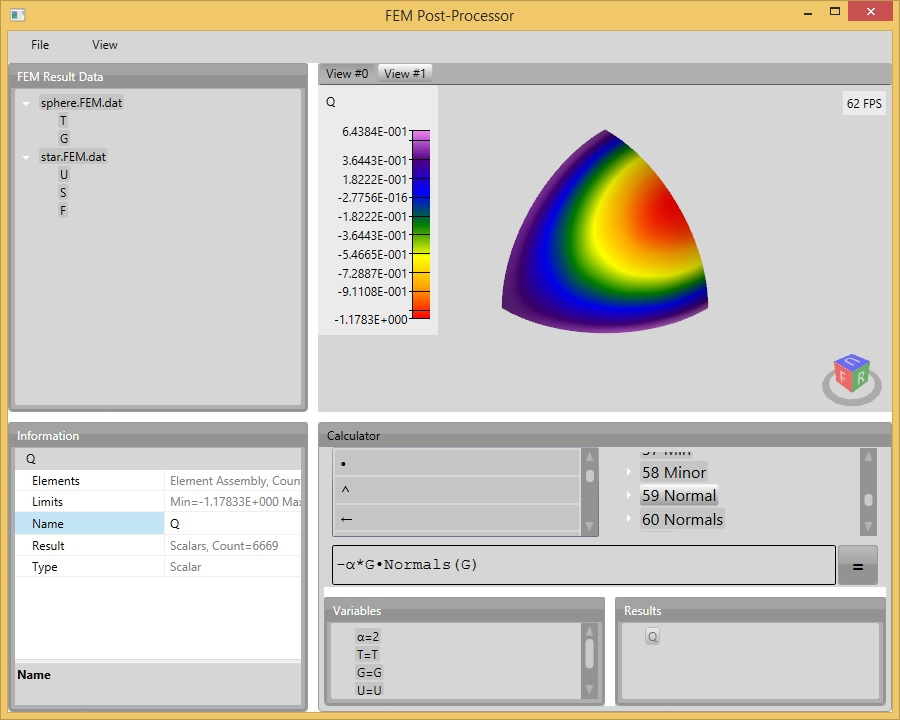Parametric Surface modeler.

SurfaceModeler.rar

Platform: .NET.

Description: Parametric surface modeler allows create and visualize complicated surfaces specified in the form of analytical equations. Equations checked for syntax errors before surface creations. The equations can be specified in one of several local coordinate system types (Cartesian, cylindrical, spherical and so on).